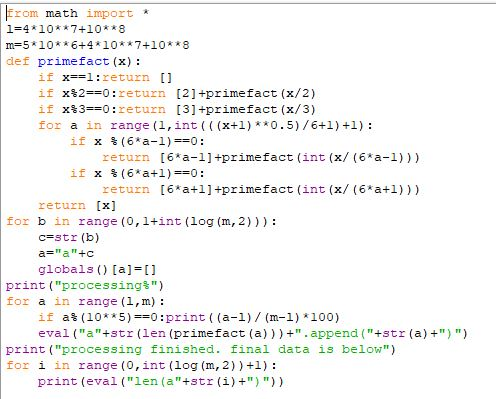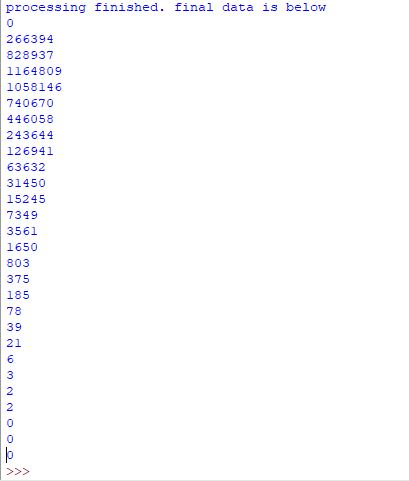# Number Of Prime factor (N.O.P.) Week 2 (Main Page)

$\large{Name\space of\space participants\space appearing}$ :

$\large{The\space program}$ :

The program have been edited a bit, so please use this new program not the one I shared before

  1 2 3 4 5 6 7 8 9 10 11 12 13 14 15 16 17 18 19 20 21 22 23 24 from math import * l=#type l here (value given to you) m=#type m here (value given to you) def primefact(x): if x==1:return [] if x%2==0:return +primefact(x/2) if x%3==0:return +primefact(x/3) for a in range(1,int(((x+1)**0.5)/6+1)+1): if x %(6*a-1)==0: return [6*a-1]+primefact(int(x/(6*a-1))) if x %(6*a+1)==0: return [6*a+1]+primefact(int(x/(6*a+1))) return [x] for b in range(0,1+int(log(m,2))): c=str(b) a="a"+c globals()[a]=[] print("processing%") for a in range(l,m): if a%(10**5)==0:print((a-l)/(m-l)*100) eval("a"+str(len(primefact(a)))+".append("+str(a)+")") print("processing finished. final data is below") for i in range(0,int(log(m,2))+1): print(eval("len(a"+str(i)+")")) 

$\large{Variable\space values}$ :

 Name of participant Value of $l$ Value of $m$
 Akela Chana $5\times10^6+3\times10^7+10^8$ $4\times10^7+10^8$
 Siddharth Chakravarty $4\times10^7+10^8$ $5\times10^6+4\times10^7+10^8$
 David Stiff $5\times10^6+4\times10^7+10^8$ $5\times10^7+10^8$
 Vinayak Srivastava $8\times10^7+10^8$ $5\times10^6+8\times10^7+10^8$
 Zakir Husain $5\times10^7+10^8$ $5\times10^6+5\times10^7+10^8$
 Jeff Giff $5\times10^6+5\times10^7+10^8$ $6\times10^7+10^8$
 Zakir Husain $6\times10^7+10^8$ $2\times10^8$

Note :

• If someone still can't complete his work then he can voluntarily ask for reduction, his work then will be transferred to me (some of it).

• If any of you will complete your work before the end of the week and wanted more then you can voluntarily ask for it.

• If you are having any doubts related to this then clear it here.

• Akela Chana, and Jeff Giff are requested to complete their previous work and submit it

• If you also want to participate (you are not a participant right now) then you can register your name for coming week (week $3$) and this week also.

• If you know a better programming language then kindly suggest.

• For more information see On Most common number of prime factor!.

• In python $a^b$ is written as :  a**b  So don't write it in this format - ''a^b'' as it will not work properly. See this for more information.

• Hopefully, we will cross $2\times10^8$ this week (if everyone do their work).

• Analysis till $2\times10^8$ will be there on the Analysis Page soon!Note by Zakir Husain
2 months, 2 weeks ago

This discussion board is a place to discuss our Daily Challenges and the math and science related to those challenges. Explanations are more than just a solution — they should explain the steps and thinking strategies that you used to obtain the solution. Comments should further the discussion of math and science.

When posting on Brilliant:

• Use the emojis to react to an explanation, whether you're congratulating a job well done , or just really confused .
• Ask specific questions about the challenge or the steps in somebody's explanation. Well-posed questions can add a lot to the discussion, but posting "I don't understand!" doesn't help anyone.
• Try to contribute something new to the discussion, whether it is an extension, generalization or other idea related to the challenge.

MarkdownAppears as
*italics* or _italics_ italics
**bold** or __bold__ bold
- bulleted- list
• bulleted
• list
1. numbered2. list
1. numbered
2. list
Note: you must add a full line of space before and after lists for them to show up correctly
paragraph 1paragraph 2

paragraph 1

paragraph 2

[example link](https://brilliant.org)example link
> This is a quote
This is a quote
    # I indented these lines
# 4 spaces, and now they show
# up as a code block.

print "hello world"
# I indented these lines
# 4 spaces, and now they show
# up as a code block.

print "hello world"
MathAppears as
Remember to wrap math in $$ ... $$ or $ ... $ to ensure proper formatting.
2 \times 3 $2 \times 3$
2^{34} $2^{34}$
a_{i-1} $a_{i-1}$
\frac{2}{3} $\frac{2}{3}$
\sqrt{2} $\sqrt{2}$
\sum_{i=1}^3 $\sum_{i=1}^3$
\sin \theta $\sin \theta$
\boxed{123} $\boxed{123}$

Sort by:

$\large{Announcement : }$ Week $3$ will be announced tomorrow morning (Indian Timing) it will go until $30$ August, $2019$. Sorry to all, for late announcement.

- 2 months ago

$\large{Announcement : }$ Week $3$ can't be announced today as I'll not be free for this. If you are interested to have more work then you may ask for it.

- 2 months, 1 week ago

Just send me any intervals you want done @Zakir Husain. Each one takes me about 10 minutes, so they're not a big deal.

- 2 months, 1 week ago

 @David Stiff Last one of this week $l=5\times10^6+5\times10^7+10^8$ $m=6\times10^7+10^8$

- 2 months, 1 week ago

Here you go!

 1 2 l = 5*10**6 + 5*10**7 + 10**8 m = 6*10**7 + 10**8 

  1 2 3 4 5 6 7 8 9 10 11 12 13 14 15 16 17 18 19 20 21 22 23 24 25 26 27 28 0 264905 826063 1164007 1057193 743436 446814 245111 127218 64224 31494 15337 7459 3512 1709 803 381 180 78 45 14 12 0 3 2 0 0 0 

- 2 months, 1 week ago

 @David Stiff $l=5\times10^6+3\times10^7+10^8$ $m=4\times10^7+10^8$

- 2 months, 1 week ago

 1 2 l = 5*10**6 + 3*10**7 + 10**8 m = 4*10**7 + 10**8 

  1 2 3 4 5 6 7 8 9 10 11 12 13 14 15 16 17 18 19 20 21 22 23 24 25 26 27 28 0 266738 830515 1164652 1058351 740344 444887 243769 126591 63470 31512 15093 7375 3548 1649 798 380 174 81 40 17 9 5 1 1 0 0 0 

- 2 months, 1 week ago

Sorry for being late @Zakir Husain

Here is the input:Here is the output:- 2 months, 2 weeks ago

- 2 months, 2 weeks ago

@Zakir Husain forgive me Sir, I am not able to complete given task. I am extremely sorry. Please cancel my participation.

- 2 months, 1 week ago

Don't worry your work is already completed, if you want you can participate in the next week, your choice.

- 2 months, 1 week ago

@Percy Jackson , are you participating ?

- 2 months, 2 weeks ago

@Zakir Husain Sir, my online classes of FIITJEE and school have both started and my schedule is becoming very hectic because I also need to get time for self studies. Also, my laptop is unable to evaluate your code because of the reason I stated. So, can you please remove me from the participants sir? I won't be able to give the output.

- 2 months, 2 weeks ago

Okay, now you are removed

- 2 months, 2 weeks ago

Do you need someone to run the missing intervals from last week? I can if you want.

- 2 months, 2 weeks ago

Yes, I was waiting for someone to ask for it. Thanks

- 2 months, 2 weeks ago

No problem. Just send me the inputs.

- 2 months, 2 weeks ago

 $l=5\times10^6+2\times10^7+10^8$ $m=3\times10^7+10^8$

- 2 months, 2 weeks ago

Here you go. Let me know if you need any more.

 1 2 l = 5*10**6 + 2*10**7 + 10**8 m = 3*10**7 + 10**8 

  1 2 3 4 5 6 7 8 9 10 11 12 13 14 15 16 17 18 19 20 21 22 23 24 25 26 27 0 268045 831905 1166995 1057042 739487 443888 242791 126268 63276 31172 15145 7330 3520 1640 788 381 176 84 32 23 5 5 0 1 1 0 

- 2 months, 2 weeks ago

Maybe not, I have my exams in 2 days, sorry :)

- 2 months, 2 weeks ago

Ran for about 10 minutes again.

 1 2 l=5*10**6 + 4*10**7 + 10**8 m=5*10**7 + 10**8 

  1 2 3 4 5 6 7 8 9 10 11 12 13 14 15 16 17 18 19 20 21 22 23 24 25 26 27 28 0 265803 828146 1164201 1058086 741915 445584 244558 127182 63543 31598 15288 7384 3489 1725 785 379 183 78 42 17 6 6 1 1 0 0 0 

- 2 months, 2 weeks ago

The code took too looooooong!

### Input

  1 2 3 4 5 6 7 8 9 10 11 12 13 14 15 16 17 18 19 20 21 22 23 24 from math import * l=8*10**7+10**8 m=5*10**6+8*10**7+10**8 def primefact(x): if x==1:return [] if x%2==0:return +primefact(x/2) if x%3==0:return +primefact(x/3) for a in range(1,int(((x+1)**0.5)/6+1)+1): if x %(6*a-1)==0: return [6*a-1]+primefact(int(x/(6*a-1))) if x %(6*a+1)==0: return [6*a+1]+primefact(int(x/(6*a+1))) return [x] for b in range(0,1+int(log(m,2))): c=str(b) a="a"+c globals()[a]=[] print("processing%") for a in range(l,m): if a%(10**5)==0:print((a-l)/(m-l)*100) eval("a"+str(len(primefact(a)))+".append("+str(a)+")") print("processing finished. final data is below") for i in range(0,int(log(m,2))+1): print(eval("len(a"+str(i)+")")) 

### Output

  1 2 3 4 5 6 7 8 9 10 11 12 13 14 15 16 17 18 19 20 21 22 23 24 25 26 27 28 29 30 31 32 33 34 35 36 37 38 39 40 41 42 43 44 45 46 47 48 49 50 51 52 53 54 55 56 57 58 59 60 61 62 63 64 65 66 67 68 69 70 71 72 73 74 75 76 77 78 79 80 81 82 processing% 0.0 2.0 4.0 6.0 8.0 10.0 12.0 14.000000000000002 16.0 18.0 20.0 22.0 24.0 26.0 28.000000000000004 30.0 32.0 34.0 36.0 38.0 40.0 42.0 44.0 46.0 48.0 50.0 52.0 54.0 56.00000000000001 57.99999999999999 60.0 62.0 64.0 66.0 68.0 70.0 72.0 74.0 76.0 78.0 80.0 82.0 84.0 86.0 88.0 90.0 92.0 94.0 96.0 98.0 processing finished. final data is below 0 262601 822631 1160527 1059007 745423 448671 246690 128167 64706 31718 15563 7457 3581 1731 802 381 190 78 43 18 7 3 4 0 1 0 0 >>> 

- 2 months, 2 weeks ago

- 2 months, 2 weeks ago

@Zakir Husain, I think I am free for 5-10 minutes just now, just tell me my l and m values. I will participate at least this week. My exams are postponed till 10th, so this week I can do.

- 2 months, 2 weeks ago

 $l=8\times10^7+10^8$ $m=5\times10^6+8\times10^7+10^8$

- 2 months, 2 weeks ago

@Zakir Husain actually my computer is too laggy and that's why it can't work for much time and so can't evaluate time taking codes. I am just searching a code so that my computer can do the work in less time. I'll require just 3-5 days. Hope you understand. :)

- 2 months, 2 weeks ago

Ok you can, but you can also break the work in small pieces so that each part will take small amount of time.

- 2 months, 2 weeks ago

- 2 months, 2 weeks ago

- 2 months, 2 weeks ago

@Yajat Shamji, will you also join?

- 2 months, 2 weeks ago

@Zakir Husain - Can I participate? If yes, please tell me what to do exactly :)

- 2 months, 2 weeks ago

Yes, Some information is given here. Will you start from today itself?

- 2 months, 2 weeks ago

Yes I understood what we are trying to do, but how can i help? @Zakir Husain

- 2 months, 2 weeks ago

You just have to run the program (you can see it in this discussion) with the values of $l,m$ special for you (it is different for different participants)

- 2 months, 2 weeks ago

So what's my L M? @Zakir Husain

- 2 months, 2 weeks ago

@Percy Jackson - also try to send the input with the output, it will make my work easier

- 2 months, 2 weeks ago

 @Percy Jackson $l=8\times10^7+10^8$ $9\times10^7+10^8$
It might take long time to run it, so if you don't have to take that much long (if your computer is just that slow), you can break it into pieces so that each piece take smaller time like this :
 $l=8\times10^7+10^8$ $5\times10^6+8\times10^7+10^8$ $l=5\times10^6+8\times10^7+10^8$ $9\times10^7+10^8$

- 2 months, 2 weeks ago

 1 2 3 4 5 processing% 0.0 1.0 =============================== Code ran out of execution time. 

- 2 months, 2 weeks ago

Don't run in the coding environment of brilliant. It will not work. Use python IDLE or any other software

- 2 months, 2 weeks ago

No. However I like these posts! Keep it up!

- 2 months, 2 weeks ago